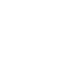### A Guide To Google Docs Date Functions

Google Docs has been my personal favorite online office program for years, I use it regularly to create documents for my college work. I also use Google spreadsheets a lot to store different types of data regarding my blog, create graphs of earnings, pageviews and then compare when required. (see example of a chart created with Google Docs)

One of the things I frequently need to use in Google spreadsheets is the date equations. I have to often call some date functions and need to create formulas so as to sort the data acording to dates. An example will make the process more clear.

Let’s say I am creating a simple spreadsheet which will contain dates from different months and the total number of pageviews on that given date. I would like to compare the increase or decrease in the percent pageviews, thus I need to know the total number of days between two dates in a Google docs spreadsheet.

There are many more examples, but let’s come directly to exploring date functions in Google Docs.

### Difference Between Two Dates

The difference between two dates is calculated using the formula DAYS360(date_1, date_2, type)The above formula returns the difference between two dates in the mm/dd/yy format. Please note that the year is in 360 days format acording to interest calculations. If Date in cell 2 is earlier than Date in cell 1, a negative number will be returned.

Application: If you have a large set of dates in one column and you want to quickly find out the difference between any two dates, this formula will be useful. Just select an entire column for displaying the result and then pick the cells containing the dates. Voila !

### Number of Workdays and Holidays Between Two Dates

This is again a very handy formula to find out the number of working days between any given pair of dates and to sort out the total number of holidays in a given period of time. The equation for finding the number of workdays and holidays is

NETWORKDAYS(start_date, end_date, holidays)### Convert a Date to Internal Serial Number

If you want to convert a date to internal serial number in a Google Docs spreadsheet; use the function =DATE(year,month,day). An example is shown below:### Convert a Binary Number to Decimal, Hexadecimal And Vice Versa

If you a large column containing binary numbers in a Google Spreadsheet and want to easily convert all of them to decimal form, use the formula BIN2DEC(number).

Simply enter the4 cell number is parenthesis e.g BIN2DEC(A4) and the binary number will be converted to it’s corresponding decimal formRelated Binary, decimal and hexadecimal functions are given below:

• Decimal to Binary – DEC2BIN(number, places)
• Decimal to hexadecimal – DEC2HEX(number, places)
• Decimal to octal – DEC2OCT(number, places)
• Hexadecimal to binary – HEX2BIN(number, places)
• Hexadecimal to decimal – HEX2DEC(number)

Remembering these formulas is also very easy – just use the syntax HEX, BIN and DEC and use “2” as the operator.

### Getting the system’s time and date in a Google Docs Spreadsheet

Sometimes. you may want to return the time and date of the computer which is using a Google Docs spreadsheet. This will be useful when you want to know when any of the shared users have added or modified valued from the spreadsheet. Use =NOW(),   as shown below:The above function does not accept arguments though. SImilarly, you can return the values of Hour, minute or month by using

• HOUR (number)
• MINUTE (number)
• MONTH (number).

There are more types of functions to consider, which ranges from logical functions, financial functions, Lookup, Math and statistical equaltions. A complete list of all functions and formulas for Google docs spreadsheets is available here.

### Top 10 Live TV Streaming Sites To Watch News, Sports, Movies### 1 comment

1.Ben Claar says:

Thanks for the article!  For anyone looking to do simple additions, subtractions, etc with dates, just try it.   (A1 + 10) will give you the date 10 days after the date in A1.  (A2-A1) will give you the difference in days between them, etc.